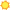# Statisticians31 matches for Statistician

 O*NET-SOC Title 1 match Alternate Occupation Titles 19 matches O*NET-SOC Description 1 match Tasks 9 matches Detailed Work Activities 1 match

## O*NET-SOC Title

• Statisticians

## Alternate Occupation Titles

• Statistical Analyst
• Statistical Consultant
• Statistical Engineer
• Statistical Program Management Division Chief
• Statistical Programmer
• Statistical Programming Director
• Statistical Reporting Analyst
• Statistician
• Analytical Statistician
• Applied Statistician
• Biological Statistician
• Clinical Statistics Manager
• Environmental Statistician
• Human Resource Statistician
• Mathematical Statistician
• Research Statistician
• Sports Statistician
• Survey Statistician
• Time Study Statistician

## O*NET-SOC Description

• Develop or apply mathematical or statistical theory and methods to collect, organize, interpret, and summarize numerical data to provide usable information. May specialize in fields such as bio-statistics, agricultural statistics, business statistics, or economic statistics. Includes mathematical and survey statisticians.

• Adapt statistical methods to solve specific problems in many fields, such as economics, biology, and engineering.
• Analyze and interpret statistical data to identify significant differences in relationships among sources of information.
• Determine whether statistical methods are appropriate, based on user needs or research questions of interest.
• Develop software applications or programming to use for statistical modeling and graphic analysis.
• Evaluate the statistical methods and procedures used to obtain data to ensure validity, applicability, efficiency, and accuracy.
• Present statistical and nonstatistical results, using charts, bullets, and graphs, in meetings or conferences to audiences such as clients, peers, and students.
• Process large amounts of data for statistical modeling and graphic analysis, using computers.
• Report results of statistical analyses in peer-reviewed papers and technical manuals.
• Report results of statistical analyses, including information in the form of graphs, charts, and tables.

## Detailed Work Activities

• Apply mathematical principles or statistical approaches to solve problems in scientific or applied fields.